凸包的几种求法 | Kyons'Blog

## 前置知识

### 凸包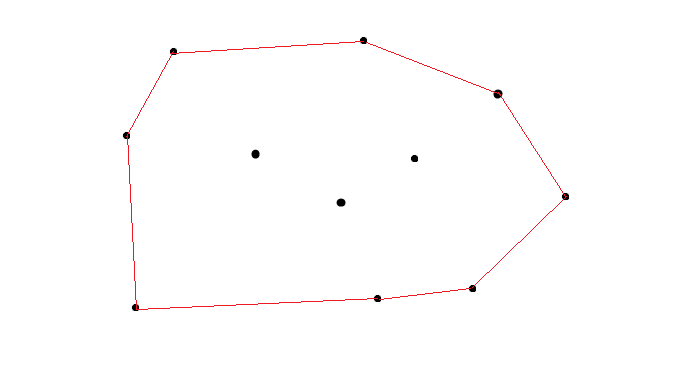### 向量旋转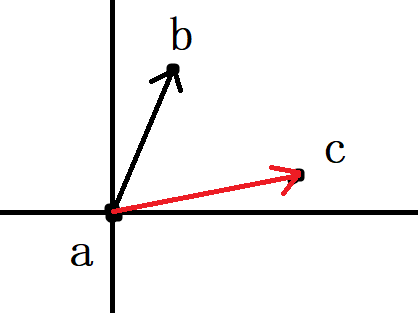$\overrightarrow{ac}$旋转为$\overrightarrow{ab}$为左旋,即逆时针旋转.

• 判断向量$\overrightarrow{ab}.x*\overrightarrow{ac}.y-\overrightarrow{ab}.y*\overrightarrow{ac}.x$的值.
• 若为正,则为$\overrightarrow{ab}$右旋变为$\overrightarrow{ac}$.
• 若为负,则为$\overrightarrow{ab}$左旋变为$\overrightarrow{ac}$.
• 若为$0$,则为共线.

## 正题

### 前篇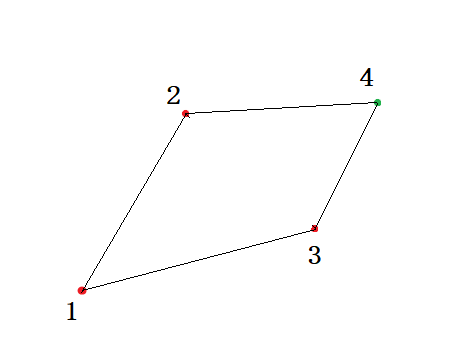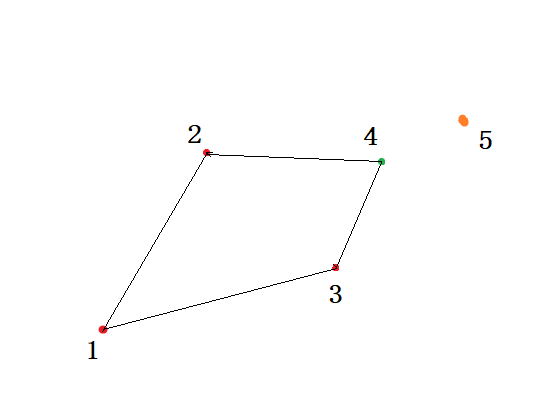1. 边$2\rightarrow4$应该断开,然后将$2\rightarrow5$连接
2. 再将$3\rightarrow4$断开.然后将$3\rightarrow5$连接
变成这样: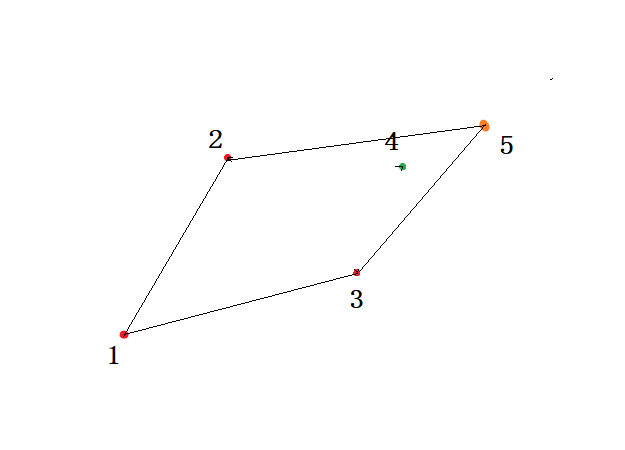我们可以简单把这个凸包划分为上半部分和下半部分.
• 对于上半部分的$1,2,4,5$
• 为什么断开$2\rightarrow4$而连接$2\rightarrow5$?
• 因为$4$在$2\rightarrow5$的内侧?
• 这是一个原因,但本质是
• 向量$\overrightarrow{2\rightarrow4}$是需要左旋才能成为$\overrightarrow{2\rightarrow5}$
• 同理可以得到,对于$3\rightarrow4$变$3\rightarrow5$
• 向量$\overrightarrow{3\rightarrow4}$是需要右旋才能成为$\overrightarrow{3\rightarrow5}$

### 中篇

• 在离线,所给的点集无序时,我们有了$O(n\log{n})$的算法.
• 当所给的点集是有序时,我们有$O(n)$的算法.

### 后篇

#### 有序的且符合简单多边形的点集

• 什么是简单多边形?

• 顶点与顶点不重合。
• 顶点不在边上。
• 边与边不相交的多边形。
• 有序是什么?

• 按照$X$,$Y$坐标排序的有序
• 按照逆时针或顺时针给出的有序,当然也阔以称之为极角排序的有序.
• 首先先看第一种排序,按照逆时针或顺时针给出的有序,(极角排序的有序).

• 当仁不让$Graham$算法

• 算法思路:
• 1.栈名$q$,栈尾指针$tail$,初始化在栈中加入最左下角的点,和第二个点
• 2.假设即将加入的点$c$
• 3.判断向量$\overrightarrow{q_{tail-1}q_{tail}}$和向量$\overrightarrow{q_{tail-1}c}$的旋转关系.
• 1).如果是$\overrightarrow{q_{tail-1}q_{tail}}$右旋变为$\overrightarrow{q_{tail-1}c}$,将栈顶元素$q_{tail}$弹出,进行步骤$3$,直到进行步骤$2)$不成立或栈内只剩$2$个元素.
• 2).如果是$\overrightarrow{q_{tail-1}q_{tail}}$左旋变为$\overrightarrow{q_{tail-1}c}$,将$c$压入栈,回到步骤$2$.
• 如果发现三点共线的情况，算法可以考虑将其视为左转或者右转。这取决于究竟只是要求凸包的边界，还是要找到在凸包边界上所有的点。
• 示意图: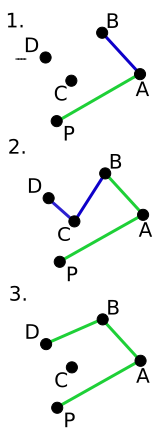• 被大家吹的很厉害的$Melkman$算法

• 援引一下$Melkman$在论文中说的:

• It is the purpose of this short article to show that a slightly modified version of their algorithm constructs, on-line, the convex hull of any simple polyline in $O(n)$ time.

• 在论文中,$Melkman$使用的是:顺时针.

• 当然,逆时针也阔以.

• 算法思路:

• 根据简单多边形的性质,我们知道边和边是不相交的.
• 1.确立一条边,使其他所有的点都在这条边的一侧
• 2.在一个双端队列的队尾放入边的两点,
• 3.再在队列头和尾都放入第三个点.
• 4.依次读入每一个点$p$,并与$q_{tail},q_{tail-1},q_{head},q_{head+1}$比较.
• 1).如果是$\overrightarrow{q_{tail-1}q_{tail}}$右旋变为$\overrightarrow{q_{tail-1}p}$,将队尾元素$q_{tail}$弹出,进行步骤$4$,直到进行步骤$1)$不成立或栈内只剩$3$个元素.
• 2).如果是$\overrightarrow{q_{tail-1}q_{tail}}$左旋变为$\overrightarrow{q_{tail-1}c}$,将$c$压入队尾,回到步骤$3$.
• 3).如果是$\overrightarrow{q_{head+1}q_{head}}$右旋变为$\overrightarrow{q_{head+1}p}$,将队首元素$q_{head}$弹出,进行步骤$4$,直到进行步骤$3)$不成立或队内只剩$3$个元素.
• 4).如果是$\overrightarrow{q_{head+1}q_{head}}$左旋变为$\overrightarrow{q_{head+1}p}$,将$p$压入队尾,回到步骤$3$.
•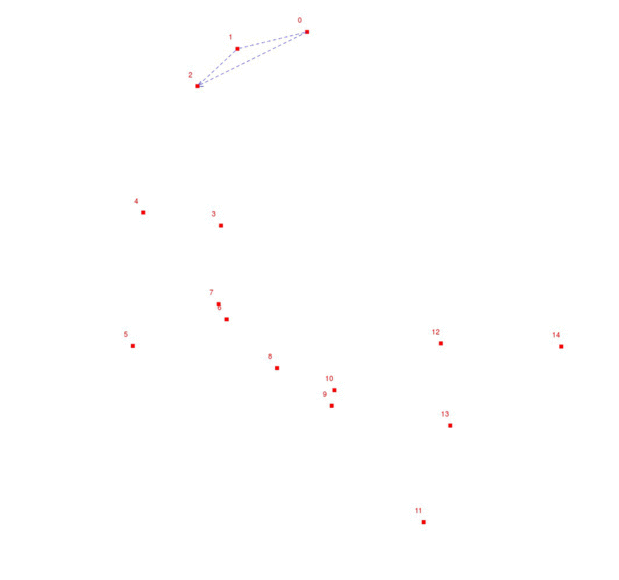• 我们来进一步理解这个算法

• 看图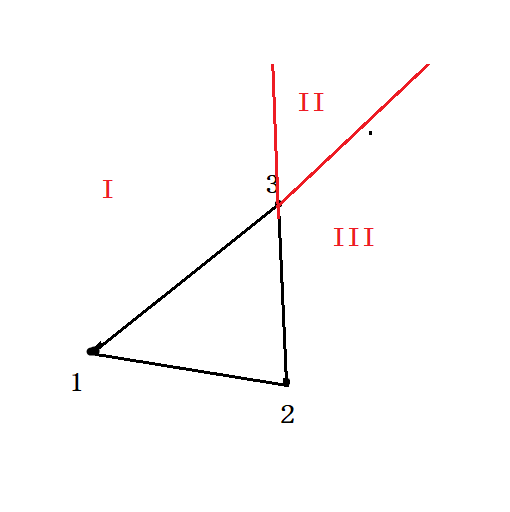• 因为所给的点是有序的,那么点只能落在$I,II,III$这三个位置.
• 在这里,我们的$q[head]$和$q[tail]$都是$3$.
• 加入点$4$便判断,在$I$,那么$head++$,然后压入$4$
• 若在$III$,那么$tail–$,压入$4$.
• 若在$II$,则$head++,tail–$,再分别压入$4$.
• 重复上述步骤,直到所有点遍历结束.
• 对于数据是按$x$从小到大,$x$相等时$y$从小到大的排列的点集

• 升级版的$Graham$算法:$Andrew$算法
• 当使用极角排序时的精度丢失又是一个折磨人的事情.
• 对于$Graham$算法,对于判断三点共线时有可能出现错误: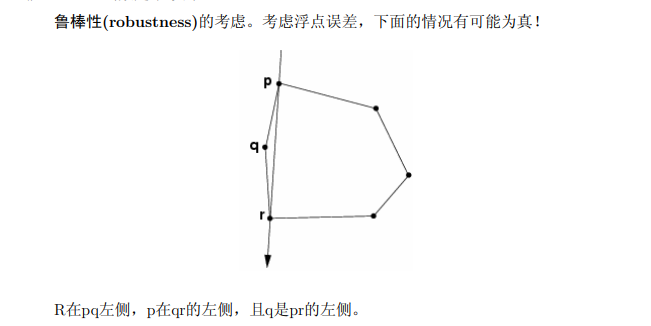• 算法思路:
• 我们结合$Graham$来看.
• 在$Andrew$算法中,数据要按照$x$坐标来排列.
• 之后,先从点$1$扫到$n$,按照$Gramham$的处理,得到上凸包
• 再从$n$扫到$1$,得到下凸包,结束.
•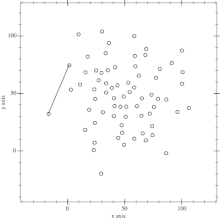## 代码

### $Andrew$算法

//删除Valine核心代码库外链调用 //调用刚下载的本地文件以加速加载速度 //这里改为从本地加载